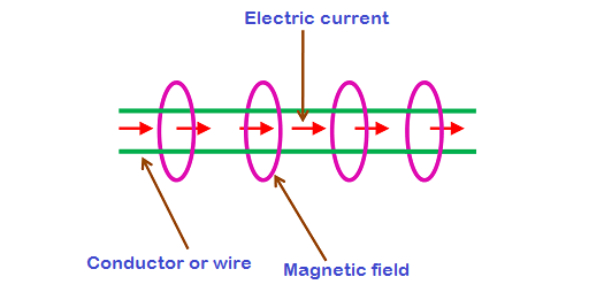# Magnetism And Electricity Quiz Questions

26 Questions | Total Attempts: 1574Settings.

• 1.
Bar magnets have two poles.  What are they?
• A.

North and south

• B.

Red and green

• C.

East and west

• D.

Red and blue

• 2.
What could you detect using a magnet and iron filings?
• A.

Electricity

• B.

Gravity

• C.

Magnetic field

• D.

• 3.
Where is the pushing a pulling force of a bar magnet strongest?
• A.

At the ends of a magnet

• B.

In the middle of the magnet

• C.

It is the same throughout the magnet

• 4.
Magnetism is a type of...
• A.

Electricity

• B.

Force

• C.

Gravity

• D.

Matter

• 5.
If magnet A can hold 3 paperclips and magnet B can hold 5 paperclips, which magnet is stronger?
• A.

Magnet A

• B.

Magnet B

• C.

They are equally strong

• D.

Magnets don't attract paperclips

• 6.
Two magnetic poles that are the same _____ each other
• A.

Attract

• B.

Repel

• C.

Confuse

• D.

Destroy

• 7.
What does repel mean?
• A.

Magnets move towards each other

• B.

Magnets move away from each other

• C.

Magnets lose their magnetic pull

• D.

• 8.
Which object would not attract a magnet?
• A.

Scissors

• B.

Nail

• C.

Water

• D.

Screw

• 9.
Which element is responsible for the magnetic force?
• A.

Iron

• B.

Aluminum

• C.

Copper

• D.

Gold

• 10.
Does distance strengthen or weaken a magnets ability to attract?
• A.

Strengthen

• B.

Weaken

• C.

Makes no difference

• 11.
Naturally occuring magnets in nature are called -
• A.

Plastics

• B.

Stones

• C.

Lodestones

• D.

Irons

• 12.
When magnets are broken into small bits ,the bits themselves can become small magnets.
• A.

True

• B.

False

• 13.
Which of these is NOT used to create a simple electromagnet?
• A.

Battery

• B.

Light bulb

• C.

Copper wire

• 14.
The negatively charged particles within atoms are
• A.

Electrons

• B.

Protons

• C.

Nucleus

• D.

Orbits

• 15.
An example of a ferromagnetic material is
• A.

Iron

• B.

Plastic

• C.

Copper

• D.

Wood

• E.

All of these

• F.

None of these

• 16.
What makes a compass work
• A.

It contains atoms

• B.

It contains charged particles

• C.

It repels magnets

• D.

It spins freely

• 17.
A stream of electrically charged particles flowing from the sun is called
• A.

A Van Allen belt

• B.

The magnetosphere

• C.

Solar wind

• D.

Magnetic field

• 18.
The region of the earth's magnetic field shaped by the solar wind is called the
• A.

Aurora

• B.

Magnetosphere

• C.

Corona

• D.

Lithosphere

• 19.
In an atom the electrons and protons are located in the nucleus.
• A.

True

• B.

False

• 20.
How can you make a metal into a temporary magnet?
• A.

Rub two magnets together

• B.

Rub a permanent magnet across the magnetic material in the same direction

• C.

Make a wish

• D.

Hook a battery up to it

• 21.
What can you say about the domains of a permanent magnet?
• A.

They are aligned in the same direction

• B.

They point in random directions

• C.

They are in the nucleus of the atom

• D.

They have electricity flowing through them

• 22.
What does a generator do?
• A.

It converts electrical energy to mechanical energy

• B.

It converts mechanical energy into electrical energy

• C.

Nothing

• D.

Attracts a magnet

• 23.
What does a motor do?
• A.

It converts electrical energy to mechanical energy

• B.

It converts mechanical energy into electrical energy

• C.

Nothing

• D.

Attracts a magnet

• 24.
How do we know that the earth has  magnetic field?
• A.

Mr. Fitz-Roy told you so

• B.

The rocks on the ocean floor leave a record of magnetic field lines

• C.

The earth does not contain any iron

• D.

We do not know if the earth has a magnetic field

• 25.
Maglev trains use magnets to elevate trains so that they never touch the tracks.  The poles of the magnets on the trains facing the poles of the magnets on the tracks must be...
• A.

The same, so they attract each other

• B.

The same, so they repel each other

• C.

Opposites, so they repel each other

• D.

Opposites, so they attract each other

Related TopicsBack to top